#DAY 20
1
Software Development

## Functor 1: 概念篇

A functor is simply something that can be mapped over

# Something (或稱 Category)

A set of values arranged in some shape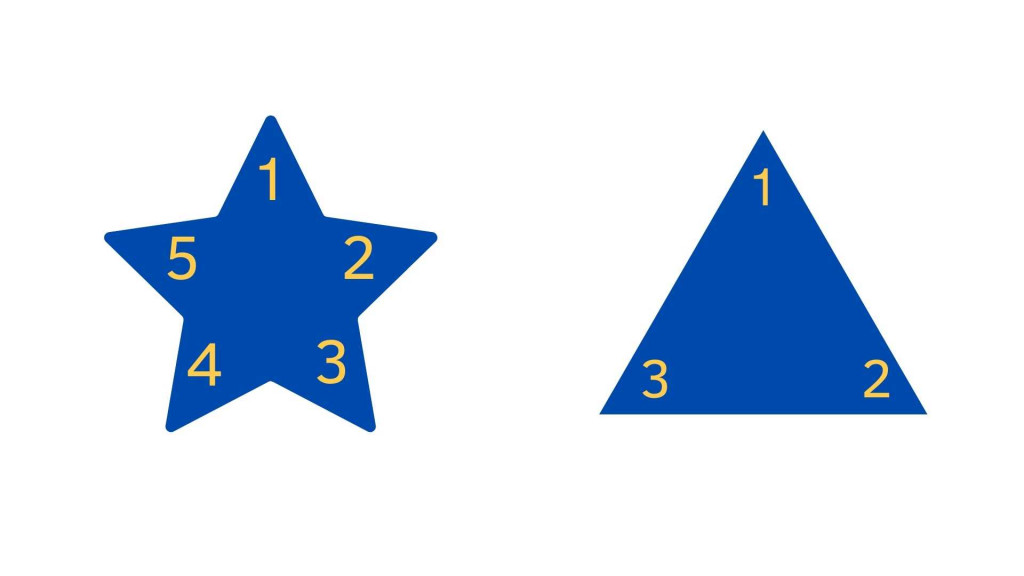↑ The values are in yellow, and the shape is in blue

An array is a set (or a collection) of values，because it’s a list of values

``````console.log([ 2, 4, 6 ].map(x => x + 3))
// => [ 5, 7, 9 ]
``````

``````// Something 1: Array
[1,2,3,4,5] // 被存放在 Array 裡的一組值

// Something 2: Object
{ age: 22, name: 'Hannah'} // 被存在物件裡的一組值

// Something 3: Single value
39 // 某個值，除了 Number 也可以是任何型別
``````

# Can be mapped over

``````[ 1, 2, 3, 4, 5 ].map(x => x + 3)); // [ 4, 5, 6, 7, 8 ]
``````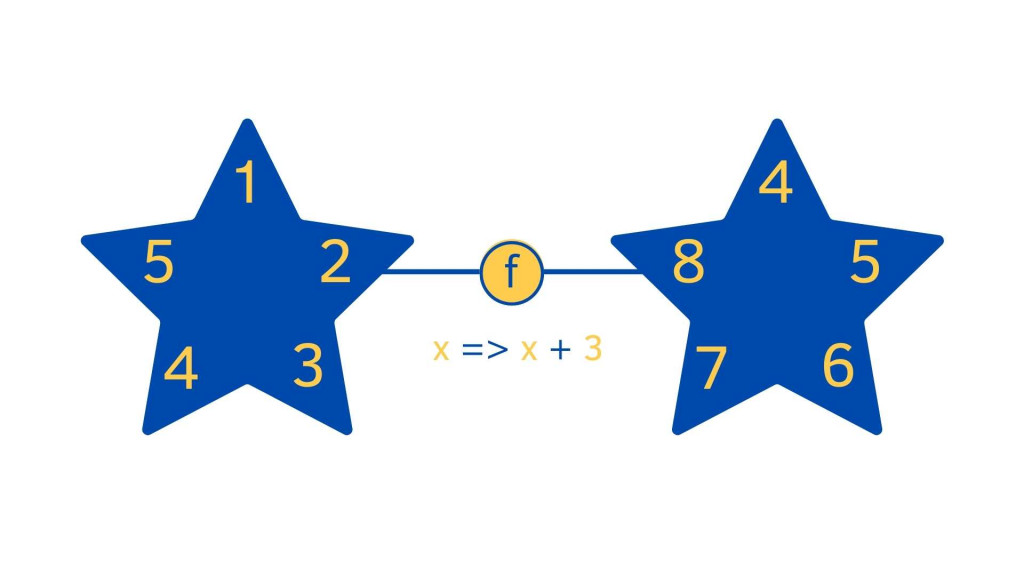list 就是 `[1, 2, 3, 4, 5, 6]` ，每一個值經過 `x => x + 3` 這個 function 後 map 到新的 list `[4, 5, 6, 7, 8]`

input map 到的 output，shape and structure 會相同

Q: 以下範例是 Functor 嗎?

``````const inputObj = { age: 22, grade: 10} // 被存在物件裡的一組值
let outputObj = {};

for (const key of Object.keys(inputObj)) {
outputObj[key] = inputObj[key] + 1
}
// { age: 23, grade: 11}
``````

A: 輸入是 `object` 輸出也是 `object`，type 相同所以是 Functor

Q: 那假如像以下輸入輸出型別不一樣呢 ?

``````[1,2,3].map(x => x.toString()) // ['1', '2', '3',]
``````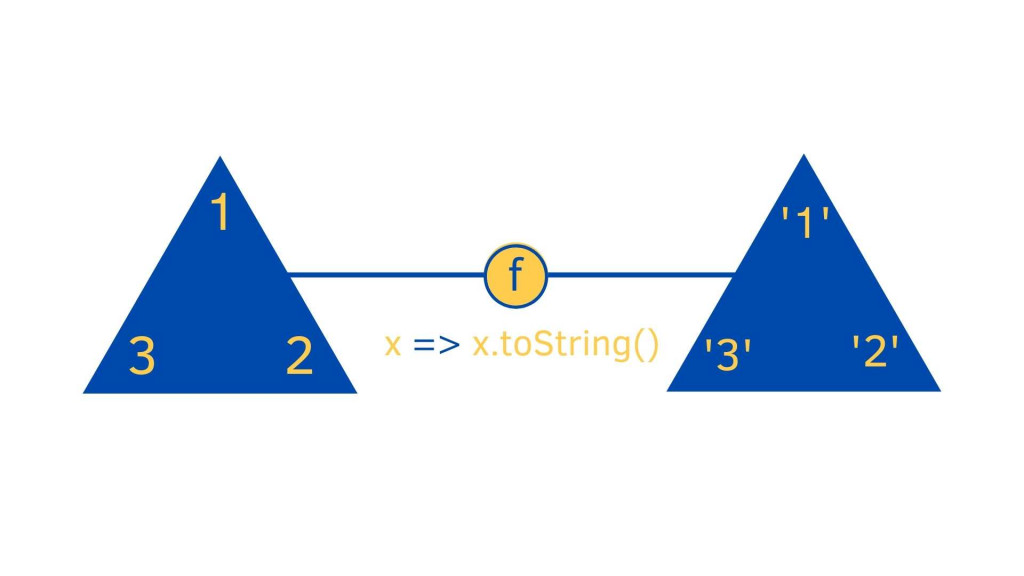A: 雖然輸入型別是 `number` ，輸出型別是 `string` 並不相同，但 type 類別相同所以也是 Functor

Q: 那若像以下星星形狀 map 到圓圈是 Functor 嗎?

``````// 範例 3，context 不同，一個是 Array 一個是 Object 所以不是
Functor[1,2,3,4,5] -> { 1: '1', 2: '2', 3: '3', 4: '4', 5: '5'}
``````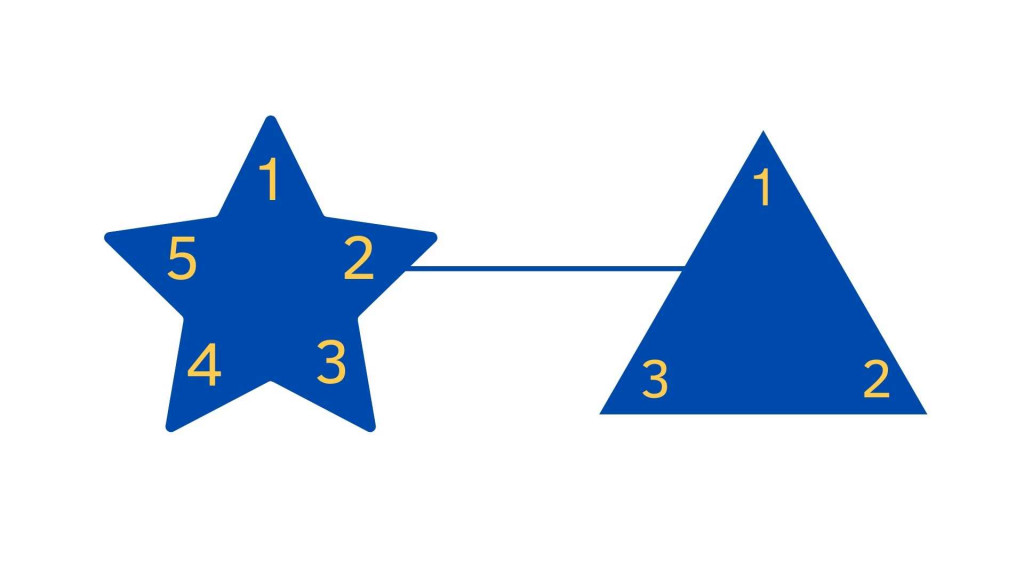A: 不是的，因為他們 type (context) 不同

Functor 除了上面說的 Array、Obj、Map、Set...也都是 Functor，另外也可以長得像下圖這樣，Number 這個值包在 NumberBox 這個 data Type 下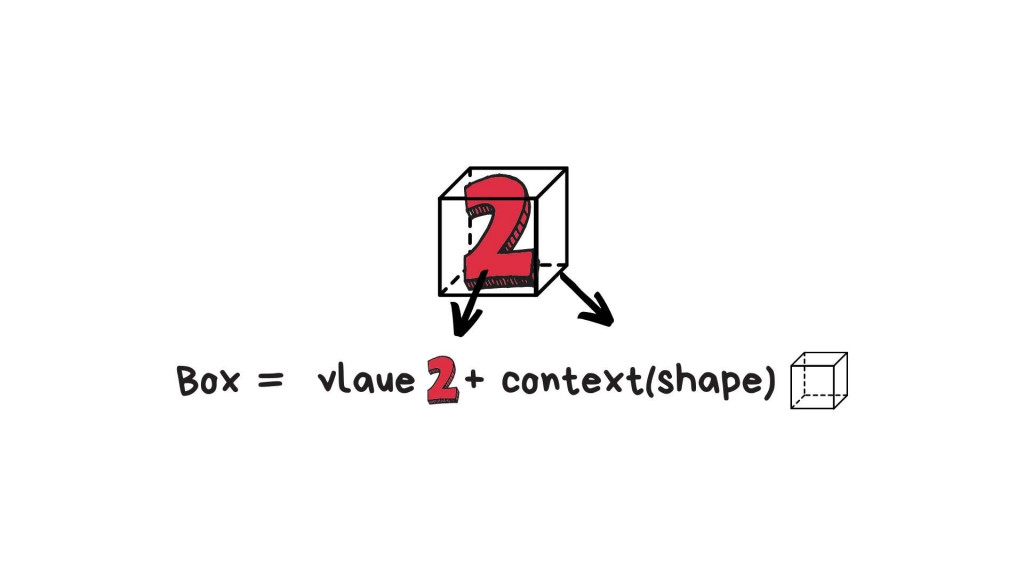``````const NumberBox = number => ({
// 這裡就是 fmap, 在 js key 通常會寫 map
fmap: fn => NumberBox(fn(number)),
value: number,
});
``````

`NumberBox` 這種 一個盒子 context (shape) 裡裝著一組數字 (Value) 也符合 Something 定義，若也滿足 `fmap` can be map over 特性也就是 Functor， 這個盒子相當重要，不過這邊就先有個記憶就好。

### 單元律

``````a.map(x => x) === a
``````

### 保存原有數據結構（可组合)

``````a.map(x => f(g(x))) === a.map(g).map(f)
``````

### 提供接口往裡面塞值

``````Effect.of = value => Effect(() => value)
``````

#### 參考文章

``````如有錯誤或需要改進的地方，拜託跟我說。

``````

### 1 則留言

2

Functor 這名詞，在不同語言中有不同的解釋，C++ 中也使用 Functor 這名詞，不過並不是這邊討論的 Functor。

``````class Functor type where
fmap :: (a -> b) -> (type a -> type b)
``````

JavaScript 中最常被稱為 Functor 的形態是 `Array`，畢竟它可以用 `map` 方法來模擬一下，先定義一個 `fmap`

``````function fmap(f) {
return array => Array.prototype.map.call(array, f);
}
``````

``````const arr2arr = fmap(x => `\${x}`);
``````

`x =>` ``\${x}``是個 `a -> b` 的函式，`a``number`，b 是 `string`，傳回的函式指定給 `arr2arr`，它是個 `type a -> type b` 的函式，只不過 type 在這邊是指 []，也就是說，若指定給 arr2arr 的引數是 [number]，傳回的會是 [string]：

``````console.log(arr2arr()); // ['1']
``````

``````console.log(arr2arr([1, 2, 3])); // ['1', '2', '3']
``````

``````const inputObj = { age: 22, grade: 10} // 被存在物件裡的一組值
let outputObj = {};

for (const key of Object.keys(inputObj)) {
outputObj[key] = inputObj[key] + 1
}
``````

``````function fmap(mapper) {
return o => {
let outputObj = {};
for(const key of Object.keys(o)) {
outputObj[key] = mapper(o[key]);
}
return outputObj;
};
}

const o2o = fmap(v => v + 1);
``````

hannahpun iT邦新手 5 級 ‧ 2020-10-07 10:05:17 檢舉